# Generate HDL Code for Simscape Models

This example uses a halfwave rectifier model to illustrate how you can develop your plant model in Simscape™ and use Simscape HDL Workflow Advisor to generate HDL code for your model.

### Why Generate HDL Code?

To perform hardware-in-the-loop (HIL) simulation with smaller timesteps and increased accuracy, you can deploy the plant models to the FPGAs on board the Speedgoat I/O modules. By using the Simscape HDL Workflow Advisor, you can generate an HDL implementation model. You can then generate HDL code for the implementation model and deploy the generated code onto the FPGA platforms. Using this capability, you can model and deploy complex physical systems in Simscape that previously took long time to model by using Simulink™ blocks.

### Simscape Example models for HDL Code generation

For HDL code generation, you can design your own Simscape algorithm or choose from a list of example models that are created in Simscape. The example models include:

• Boost Converter

• Bridge Rectifier

• Buck Converter

• Halfwave Rectifier

• Three Phase Rectifier

• Two Level Converter Ideal

• Two Level Converter Igbt

All examples are prefixed with `sschdlex` and postfixed with `Example`. For example, to open the Boost Converter model, in the MATLAB™ command window, enter:

```load_system('sschdlexBoostConverterExample') open_system('sschdlexBoostConverterExample/Simscape_system') ```

### Restrictions for HDL Code Generation from Simscape Models

HDL Coder™ does not support code generation from Simscape networks that contain:

• Events

• Mode charts

• Delays

• Runtime parameters

• Periodic sources

• Simscape™ Multibody™ blocks

• Simscape Electrical Specialized Power Systems blocks

• Nonlinear and time-varying Simscape blocks. Time-varying blocks include blocks such as Variable Inductor and Variable Capacitor.

### Guidelines for Modeling Simscape for HDL Compatibility

1. Create a Simscape model by using switched linear blocks. Switched linear blocks are blocks such as diodes and switches. These blocks are defined by a linear relationship such as V = IR where R can switch between two or more values depending on the state of the diodes or switches. Add Simulink-PS Converter blocks at the input ports and PS-Simulink Converter blocks at the output ports.

2. Configure the solver options for HDL code generation by using a Solver Configuration block. In the block parameters of this block:

• Select Use local solver.

• Use `Backward Euler` as the Solver type.

• Specify a discrete sample time, Ts.

3. Enclose the blocks inside a Subsystem and provide the test inputs.

4. Configure the model for HDL code generation by running the `hdlsetup` function. hdlsetup configures the solver settings such as using a fixed-step solver, specifies the simulation start and stop times, and so on. To run the command for your `current_model`:

```hdlsetup('current_model') ```

5. Verify Simscape model compatibility by using the `simscape.findNonlinearBlocks` function. This function detects nonlinear blocks in your Simscape model. Provide the path to your Simscape model as an argument to this function. It returns the names of nonlinear blocks.

For example: To verify presence of nonlinear blocks in Half Wave Rectifier Model, in the MATLAB command window, enter:

```simscape.findNonlinearBlocks('sschdlexHalfWaveRectifierExample') ```

### The Halfwave Rectifier Model

To open the half-wave rectifier model, in the MATLAB Command Window, enter:

```open_system('sschdlexHalfWaveRectifierExample') ```

Save this model locally as `HalfWaveRectifier_HDL` to run the workflow.

```load_system('HalfWaveRectifier_HDL') open_system('HalfWaveRectifier_HDL/Simscape_system') set_param('HalfWaveRectifier_HDL', 'SimulationCommand', 'update'); ```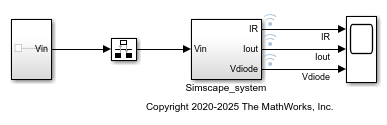The Simscape model uses switched linear blocks such as Diodes and Resistors to model the design. The model has Simulink-PS Converter blocks at the input port and PS-Simulink converter blocks at the output ports. To verify that you configured the solver settings correctly, open the Solver Configuration block.

At the top level of the model, you see a `Simscape_system` block that models the half-wave rectifier algorithm. The model accepts a Sine Wave input, uses a Rate Transition block to discretize the continuous time input, and has a Scope block that calculates the output. To see the input stimulus and the output from the model, connect the Sine Wave input to the Scope block.

```open_system('HalfWaveRectifier_HDL') ```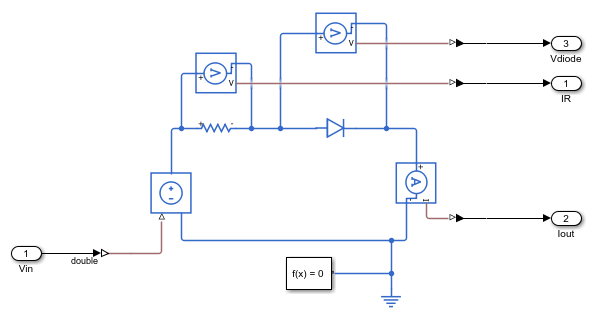To configure the half-wave rectifier model for HDL compatibility, in the MATLAB command window, enter:

```hdlsetup('HalfWaveRectifier_HDL') ```
```### The configuration parameter values use the recommended settings for HDL code generation and does not need any modification as a part of hdlsetup. Please refer to <a href="matlab:helpview(fullfile(docroot, 'hdlcoder', 'helptargets.map'), 'msg_hdlsetup_function')">hdlsetup</a> document for best practices on model settings. ```

### Simulate and Verify Functionality of Simscape Algorithm

To see the simulation results, simulate the model and then open the Scope block.

```sim('HalfWaveRectifier_HDL') ```

This figure shows simulation results with the sine wave input and the outputs from `Simscape_system`.### Open Simscape HDL Workflow Advisor

To generate an HDL implementation model from which you can generate code, use the Simscape HDL Workflow Advisor. To open the Advisor, run this command:

```sschdladvisor('HalfWaveRectifier_HDL') ```
```### Running Simscape HDL Workflow Advisor for <a href="matlab:(HalfWaveRectifier_HDL)">HalfWaveRectifier_HDL</a> ```

### Run Simscape HDL Workflow Advisor

To run the workflow, in the Simscape HDL Workflow Advisor, right-click the Generate implementation model task and select Run to Selected Task.If the task passes, you see a link to the implementation model.

In some cases, your Simscape algorithm may not be compatible for generating an implementation model using the Simscape HDL Workflow Advisor. In such cases, running certain tasks in the Advisor can result in the task to fail. To learn how you can make it HDL compatible, see

### Open HDL Implementation Model

To see the implementation model, in the Generate implementation model task, click the link.

```open_system('gmStateSpaceHDL_HalfWaveRectifier_HDL') set_param('gmStateSpaceHDL_HalfWaveRectifier_HDL','SimulationCommand','Update') ```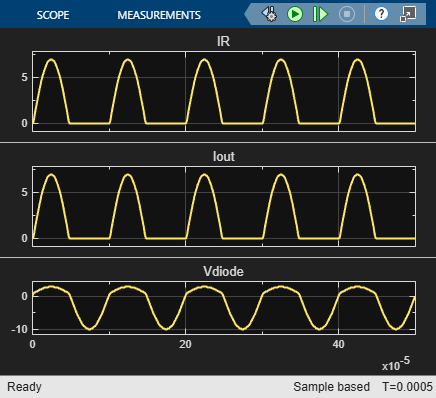The model contains two subsystems. The Subsystem block contains the Simscape algorithm that you modeled. From and Goto blocks inside this Subsystem provide the same Sine Wave input to the HDL Subsystem.

The HDL Subsystem models the state-space representation that you generated from the Simscape model. The ports of this Subsystem use the same name as the Simulink-PS Converter and PS-Simulink Converter blocks in your original Simscape model. If you navigate inside this Subsystem, you see several delays, adders, and Matrix Multiply blocks that model the state-space equations.

```open_system('gmStateSpaceHDL_HalfWaveRectifier_HDL/HDL Subsystem/HDL Algorithm') ```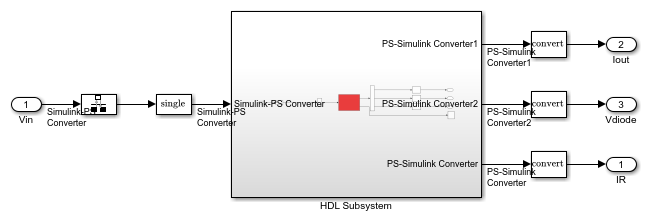To simulate the HDL Implementation model, enter this command:

```sim('gmStateSpaceHDL_HalfWaveRectifier_HDL') ```

Open the scope block to view results.The simulation results from the HDL implementation model matches that of the original plant model. Therefore, we can verify that the plant simulation model is correctly transformed into an HDL implementation model.

HDL code is generated for the `HDL Subsystem` block inside this model.

### Generate HDL Code and Validation Model

The HDL model and subsystem parameter settings are saved using this command:

```hdlsaveparams('gmStateSpaceHDL_HalfWaveRectifier_HDL'); ```
```%% Set Model 'gmStateSpaceHDL_HalfWaveRectifier_HDL' HDL parameters hdlset_param('gmStateSpaceHDL_HalfWaveRectifier_HDL', 'FloatingPointTargetConfiguration', hdlcoder.createFloatingPointTargetConfig('NativeFloatingPoint' ... , 'LatencyStrategy', 'MIN') ... ); hdlset_param('gmStateSpaceHDL_HalfWaveRectifier_HDL', 'MaskParameterAsGeneric', 'on'); hdlset_param('gmStateSpaceHDL_HalfWaveRectifier_HDL', 'Oversampling', 60); % Set SubSystem HDL parameters hdlset_param('gmStateSpaceHDL_HalfWaveRectifier_HDL/HDL Subsystem', 'FlattenHierarchy', 'on'); hdlset_param('gmStateSpaceHDL_HalfWaveRectifier_HDL/HDL Subsystem/HDL Algorithm/Mode Selection/Generate Mode Vector', 'Architecture', 'MATLAB Datapath'); ```

The model uses single data types and generates HDL code in native floating-point mode. Floating-point operators can introduce delays. Because the design contains feedback loops, for the model transformation advisor to allocate enough delays for the operators inside the feedback loops, the model uses clock-rate pipelining in conjunction with a large value for the Oversampling factor. An Oversampling factor of 100 and the clock-rate pipelining optimization is saved on this model.

Before you generate HDL code, it is recommended to enable generation of the validation model. The validation model compares the output of the generated model after code generation and the original model. To learn more, see Generated Model and Validation Model.

Run these commands to save validation model generation settings on your Simulink model:

```HDLmodelname = 'gmStateSpaceHDL_HalfWaveRectifier_HDL'; hdlset_param(HDLmodelname, 'TargetDirectory', 'C:/Temp/hdlsrc'); hdlset_param(HDLmodelname, 'GenerateValidationModel', 'on'); ```

To generate HDL code, run this command:

```makehdl('gmStateSpaceHDL_HalfWaveRectifier_HDL/HDL Subsystem'); ```

The generated HDL code and validation model are saved in `C:/Temp/hdlsrc` directory. The generated code is saved as `HDL_Subsystem_tc.vhd`. To open the validation model, click the link to `gm_gmStateSpaceHDL_HalfWaveRectifier_HDL_vnl.slx` in the code generation logs in the Command Window.

Open the Compare block at the output of `HDL Subsystem_vnl` Subsystem of the validation model. Then, open the `Assert_Out1` block. To see the simulation results after HDL code generation, open the `Compare: Out1` Scope block:The top graph represents the output of the generated model, and the middle graph represents the output of the implementation model. Since the output generated by both the models are exactly matching, the error between them is zero, which is represented in the last graph.

### Optimize the HDL Algorithm

Before you generate HDL code for the HDL Subsystem, you can optimize the algorithm by using optimizations in HDL Coder. The optimizations save resources or improve the timing of your design on the target FPGA device.

For example, to save resources on the target FPGA device, you can use the resource sharing optimization. Resource sharing is an area optimization that identifies multiple functionally equivalent resources and replaces them with a single. The data is time-multiplexed over the shared resource to perform the same operations. See Resource Sharing.

In the HDL implementation model, you can share the masked subsystem blocks that perform state updates and compute the output. To share the subsystems, specify a SharingFactor on the subsystems.

```Multiplyinputsubsys = 'gmStateSpaceHDL_HalfWaveRectifier_HDL/HDL Subsystem/HDL Algorithm/State Update'; Multiplystatesubsys = 'gmStateSpaceHDL_HalfWaveRectifier_HDL/HDL Subsystem/HDL Algorithm/Output'; open_system(Multiplyinputsubsys) ```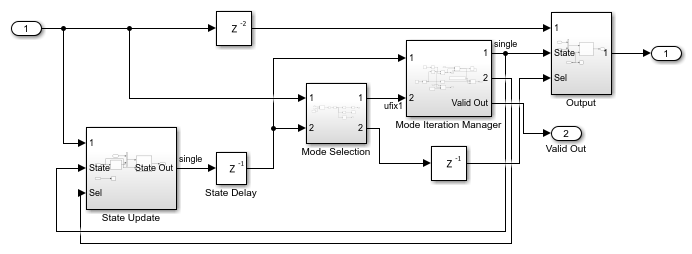```open_system(Multiplystatesubsys) ```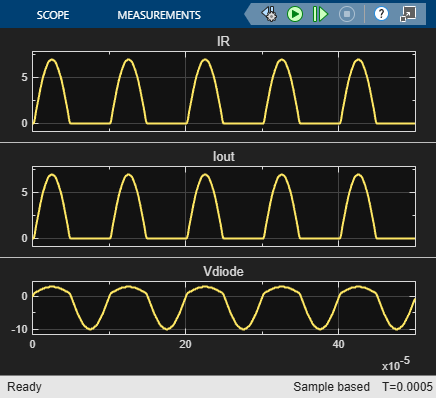To share these subsystems and generate HDL code:

1. Specify a SharingFactor of 2 on the Multiply Input and Multiply State subsystems.

```hdlset_param([Multiplyinputsubsys '/Multiply Input'],'SharingFactor', 2) hdlset_param([Multiplystatesubsys '/Multiply State'],'SharingFactor', 2) ```

2. Enable generation of the resource utilization report and the optimization report.

```hdlset_param(HDLmodelname, 'resourcereport', 'on', 'optimizationreport', 'on') ```

3. Generate HDL code for the HDL Subsystem block in the implementation model.

```makehdl('gmStateSpaceHDL_HalfWaveRectifier_HDL/HDL Subsystem'); ```

When you generate code, HDL Coder opens a Code Generation report. To see the status of the resource sharing optimization, click the Streaming and Sharing section of the report.